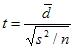# Paired Sample T-Test Illustration

Paired t-test is a statistical technique/experimental method that is used to compare two population means in the case of two samples that are correlated.  Paired sample t-test is used in ‘before-after’ studies, or when the samples are the matched pairs, or when it is a case-control study.

For example, if we give training to a company employee and we want to know whether or not the training had any impact on the efficiency of the employee, we could use the paired sample test.  We collect data from the employee on a seven scale rating, before the training and after the training.  By using the paired sample t-test, we can statistically conclude whether or not training has improved the efficiency of the employee.  In medicine, by using the paired sample t-test, we can figure out whether or not a particular medicine will cure the illness.
Steps:
1. Set up hypothesis: We set up two hypotheses.  The first is the null hypothesis, which assumes that the mean of two paired samples are equal. The second hypothesis will be an alternative hypothesis, which assumes that the means of two paired samples are not equal.
2. Select the level of significance: After making the hypothesis, we choose the level of significance.  In most of the cases, significance level is 5%,  (in medicine, the significance level is set at 1%).
3. Calculate the parameter: To calculate the parameter we will use the following formula:Where d bar is the mean difference between two samples, s² is the sample variance, n is the sample size and t is a paired sample t-test with n-1 degrees of freedom. An alternate formula for paired sample t-test is:4. Testing of hypothesis or decision making: After calculating the parameter, we will compare the calculated value with the table value.  If the calculated value is greater than the table value, then we will reject the null hypothesis for the paired sample t-test.  If the calculated value is less than the table value, then we will accept the null hypothesis and say that there is no significant mean difference between the two paired samples.
Assumptions:
1. Only the matched pairs can be used to perform the test.
2. Normal distributions are assumed.
3. The variance of two samples is equal.
4. Cases must be independent of each other.